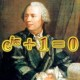# Inscribed Square Problem

Here’s another fun little geometry problem from MathChallenges.net. I found a different solution then what they gave. Let me know how you solve it.

## 3 thoughts on “Inscribed Square Problem”

1.Jeffrey He on said:

This is a very intresting problem. We used to do some of these problems in Geometry with Mrs. Polishcuck.
—————————————————
What I did was…

So let’s name the sides of the square “s”.

and we know that a^2 and b^2 gets you the hypotunse.

We also know that the height of the mini top triangle is (a-s). and the width of the bottom right triangle is (b-s).

By finding and adding the hypotunse of these two mini triangles, you get the hypotunese of the big triangle.

In math terms…..
——————————————————
*Note: Sq( ) stands for square root.

sq(a^2 + b^2) = sq((a-s)^2 + s^2) + sq((b-s)^2 + s^2)

If you symplify it, you should get the answer….

I know that it gets really messy, and that this is probally not the most practical approach…but it should be correct.

Sorry that I didn’t have time to find out the final answer…since I had a english essay to finish. ^_^

but theoratically this approach should work despite the superlong squareroots and FOIL-ing that you have to do. XD

2.qaddr on said:$\\ \frac{1}{2}ab = g^2 + \frac{1}{2}(b-g)(g) + \frac{1}{2}g(a-g) \\ ab = 2g^2 + (b-g)g + (a-g)g \\ ab = 2g^2 + (b + a - 2g)g \\ ab = 2g^2 + gb + ga - 2g^2 \\ ab = gb + ga \\ \frac{ab}{a+b} = g$
•Mr. Chase on said:
(And great work with the$LaTeX$ !)